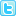# Abstract

## Prediction of global solar radiation using relative humidity, maximum temperature and sunshine hours in Uyo, in the Niger Delta Region, Nigeria

In this paper, models are developed with regression equations to predict the monthly global solar radiation future time based on measured air temperature, relative humidity and sunshine hour values between 1991-2007 for Uyo, in Niger Delta Region, Nigeria. Using the Angstrom model as the basis, other regression equations were developed by modifying Angstrom equation. The value of correlation coefficient (r) and value of the mean bias error (MBE), mean square error (RMSE) and mean percentage error root (MPE) were determined for the equation. The equation with the highest value of correlation coefficient (r ) and at least values of RMSE, MBE and MPE is given as 100 1.387 1.592 0.045 0.004 0 R Tm S S Ho Hp = + - + . The results obtained show a remarkable agreement between the measured and the predicted values using different models. The developed model can be used for the prediction of global solar radiation on horizontal surfaces for Uyo in Niger Delta

Author(s): Ituen, Eno. E., Esen, Nsikan. U., Nwokolo, Samuel. C. and Udo, Ema. G.

Abstract | PDF

Share this• ### 20th Annual Meet on Pharmaceutical Sciences

Bangkok, Thailand
###### Abstracted/Indexed in
• Chemical Abstracts Service (CAS)
• Index Copernicus